RS Aggarwal Class 10 Solutions Chapter 13 - Constructions Ex 13A (13.1)

RS Aggarwal Class 10 Chapter 13 - Constructions Ex 13A (13.1) Solutions Free PDF

Q.1: Draw a circle of radius 3cm. From a point P, 7cm away from the center of the circle draw two tangents to the circle. Also, measure the lengths of the tangents.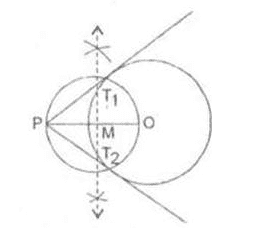Steps of Construction:

Step 1: Draw a circle of radius 5 cm with center O.

Step 2: A point P at a distance of 8cm from O is taken.

Step 3: A right bisector of OP meeting OP at M is drawn.

Step 4: With center M radius OM a circle is drawn intersecting the previous circle at T1 and T2

Step 5: Join PT1 and PT2

PT1 and PT2 and the required tangents, Measuring PT1 and PT2

We find, PT1 = PT2 = 6.2 cm

Q.2: Draw two tangents to a circle of radius 3.5 cm from a point P at a distance of 6.2cm from its center.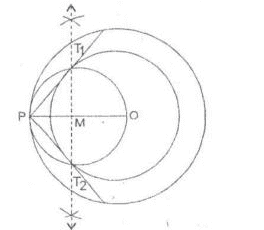Steps of construction:

Step 1: Two concentric circles with centre O and radii 4cm and 6 cm are drawn.

Step 2: A point P is taken on outer circle and O, P are joined.

Step 3: A right bisector of OP is drawn bisecting OP at M.

Step 4: With centre M and radius OM a circle is drawn cutting the inner circle at T1 and T2

Step 5: Join PT1 and PT1

PT1 and PT2 are the required tangents. Further PT1 = PT2 = 4.8 cm.

Q.3:Draw a circle of radius 3.5cm. Take two points A and B on one of its extended diameter, each at a distance of 5cm from its centre. Draw tangents to the circle from each of these points A and B.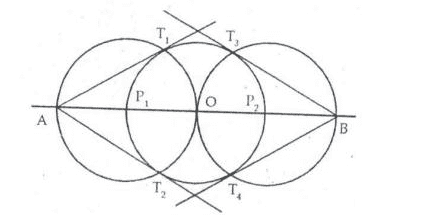Steps of construction:

Step 1: Draw a circle with center O and radius 3.5 cm

Step 2: the diameter P1P2 is extended to the points A and B such that AO = OB = 7 cm.

Step 3: With center P1 and radius 3.5 cm draw a circle cutting the first circle at T1 and T2

Step 4: Join AT1 and AT2

Step 5: With center P2 and radius 3.5 cm draw another circle cutting the first circle at T3 and T4

Step 6: Join BT3 and BT4. Thus AT1, AT2 and BT3, BT4 are the required tangents to the given circle from A and B.

Q.4:Draw a circle with centre O and radius 4cm. Draw any diameter AB of this circle. Construct tangents to the circle at each of the two end points of the diameter AB.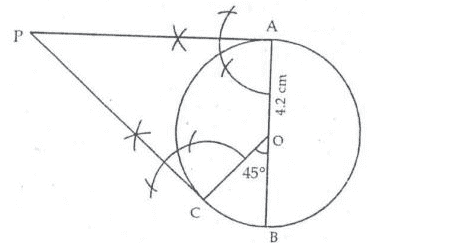Steps of Construction:

Step 1: A circle of radius 4.2 cm at center O is drawn.

Step 2: A diameter AB is drawn.

Step 3: With OB as the base, an angle BOC of 45o is drawn.

Step 4: At A, a line perpendicular to OA is drawn.

Step 5: AT C, a line perpendicular to OC is drawn.

Step 6: These lines intersect with each other at P.

PA and PC are the required tangents.

Q.5:Draw a circle with the help of a bangle. Take any point P outside the circle. Construct the pair of tangents from the point P to the circle.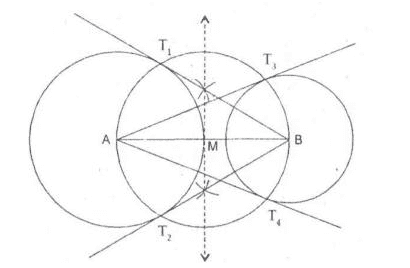Steps of Construction:

Step 1: A line segment AB = 8, 5 cm is drawn.

Step 2: Draw a right bisector of AB which meets AB at M.

Step 3: With M as center AM as radius a circle is drawn intersecting the given circles at T1, T2, T3 and T4

Step 4: Join AT3, AT4 and BT1 and BT2

Thus AT3, AT4, BT1, BT2 are the required tangents.

Q.6: Draw a line segment AB of length 8cm. Taking A as the centre, draw a circle of radius 4cm and taking B as the center, draw another circle of radius 3 cm. Construct tangents to each circle from the center of the other circle.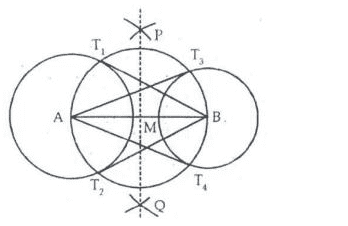Step 1: Draw a line segment AB = 7cm

Step 2: Taking A as center and radius 3 cm, a circle is drawn.

Step 3: With center B and radius 2.5 cm, another circle is drawn.

Step 4: With center A and radius more than ½ AB, arcs are drawn of both sides of AB.

Step 5: With center B and the same radius [as in step 4] arcs are drawn on both sides of AB intersecting previous arcs at P and Q.

Step 6: Join PQ which meets AB at M.

Step 7: With center M and radius AM, a circle is drawn which intersects circle with center A at T1 and T2 and the circle with the center B at T3 and T4.

Step 8: Join AT3, AT4, BT1 and BT2

Thus AT3, AT4, BT1 and BT2 are the required tangents.

Q.7:Draw a circle of radius 4.32cm. Draw a pair of tangents to this circle inclined to each other at an angle of 45°.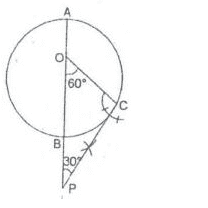Steps of construction:

Step 1: A circle of radius 3 cm with center O is drawn.

Step 2: A radius OC is drawn making an angle of 60o with the diameter AB.

Step 3: At C, $\angle$ OCP = 90o is drawn.

CP is required tangent.

Q.8:Write the steps of construction for drawing a pair of tangents to a circle of radius 3cm, which are inclined to each other at an angle of 60°.

Steps of Construction:

Step 1: Draw a circle with center O and radius 3 cm.

Step 2: Draw any diameter AOB of the circle.

Step 3: Construct ∠BOC = 60° such that radius OC cuts the circle at C.

Step 4: Draw AM perpendicular to AB and CN perpendicular to OC. Suppose AM and CN intersect each other at P.

Here, AP and CP are the pair of tangents to the circle inclined to each other at an angle of 60°.

Q.9:Draw a circle of radius 3cm. Draw a tangent to the circle making an angle of 30° with a line passing through the center.

Steps Of construction:

Step 1: Draw a circle with center O and radius 3 cm.

Step 2: Draw radius OA and produce it to B.

Step 3: Make ∠AOP = 60°.

Step 4: Draw PQ perpendicular to OP, meeting OB at Q.

Step 5: Then, PQ is the desired tangent, such that ∠OQP = 30°.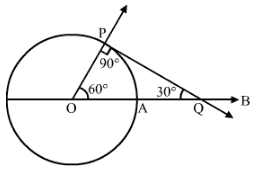Q.10:Construct a tangent to a circle of radius 4cm from a point on the concentric circle of radius 6cm and measure its length. Also, verify the measurement by actual calculation.

Steps of Construction:

Step 1: Mark a point O on the paper.

Step 2: With 0 as center and radii 4 cm and 6 cm, draw two concentric circles.

Step 3: Mark a point P on the outer circle.

Step 4: Join OP.

Step 5: Draw the perpendicular bisector XY of OP, cutting OP at O.

Step 6: Draw a circle with O as center and radius OQ (or P0), to intersect the inner circle in points, T and T’.

Step 7: Join PT and PT’.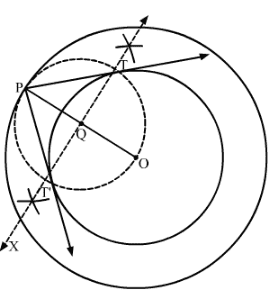Here, PT and PT’ are the required tangents.

PT = PT’ = 4.5cm (Approx)

Practise This Question

Which of the following elements does not lose an electron easily?